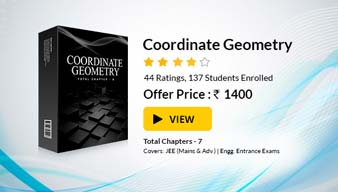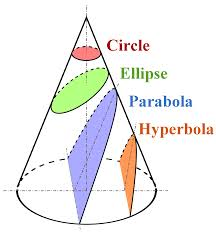×#### Thank you for registering.

One of our academic counsellors will contact you within 1 working day.

Click to Chat

1800-1023-196

+91-120-4616500

CART 0

• 0

MY CART (5)

Use Coupon: CART20 and get 20% off on all online Study Material

ITEM
DETAILS
MRP
DISCOUNT
FINAL PRICE
Total Price: Rs.

There are no items in this cart.
Continue Shopping```Co-ordinate Geometry

Table of Content

The Coordinate Plane

The Midpoint of a Line Joining Two Points

The Gradient of a Line Joining Two Points

Parallel and Perpendicular Lines

Related ResourcesCo-ordinate Geometry is a method of analyzing geometrical shapes. It is one of the most scoring topics of the mathematics syllabus of IIT JEE and other engineering exams. Besides calculus, this is the only topic that can fetch you maximum marks. It is a vast topic and can further be divided into various parts like:

Circle

Parabola

Ellipse

Hyperbola

Straight Lines

All these topics hold great importance from examination point of view but the Straight Line and the Circle are the most important. These topics together fetch maximum questions in the JEE and moreover they are a pre requisite to conic sections as well.

The Coordinate Plane

In Coordinate geometry, points are placed on the coordinate plane. The horizontal line is the x-axis while the vertical line is the y-axis. The point where they cross each other is called the origin.

A point's location on the plane is given by two numbers, the first tells where it is on the x-axis and the second tells where it is on the y-axis. Together, they define a single, unique position on the plane.

The figure given belo illustrates the cooordinate axis. The position of a point is given by an ordered pair in which the order is important as the first in the pair always stands for the x coordinate. Sometimes these are also referred to as the rectangular coordinates.We have listed some of the important facts here, but the rest have been covered in detail in the later sections.

The Midpoint of a Line Joining Two Points

The midpoint of the line joining the points (x1, y1) and (x2, y2) is:

[½(x1 + x2), ½(y1 + y2)

Illustration:

Find the coordinates of the midpoint of the line joining (11, 2) and (3, 4).

Midpoint = [½(11+ 3), ½(2 + 4)] = (7, 3)

The Gradient of a Line Joining Two Points

The gradient of a line joining two points is given by

(y2-y1) / (x2-x1)

Parallel and Perpendicular Lines

Two parallel lines have the same gradient while if two lines are perpendicular then the product of their gradient is -1.

Example

a) y = 4x + 1
b) y = -1/4 x + 12
c) ½y = 2x - 3

The gradients of the lines are 4, -1/4 and 4 respectively. Hence, as stated above lines (a) and (b) are perpendicular, (b) and (c) are perpendicular and (a) and (c) are parallel.

Watch this Video for more reference

Figure right hand side shows the various topics included in the conic sections:Generally, conic section includes ellipse, parabola and hyperbola but sometimes circle is also included in conic sections. Circle can actually be considered as a type of ellipse. When a cone and a plane intersect and their intersection is in the form of a closed curve, it leads to the formation of circle and ellipse. As it is visible from the figure above, the circle is obtained when the cutting plane is parallel to the plane of generating circle of the cone. Similarly, in case the cutting plane is parallel to one generating line of the cone, the resulting conic is unbounded and is called parabola. The last case in which both the halves of the cone are intersected by the plane, produce two distinct unbounded curves called hyperbola.

Conic section is a vital organ of coordinate geometry. It is easy to gain marks in this section as there are some standard questions asked from this section so they can be easily dealt with. Some topics no doubt are difficult but can be mastered with continuous practice. All these sections including circles, straight lines, ellipse, parabola and hyperbola have been discussed in detail in the coming sections.

Illustration:

If the circle x2 + y2 + 2x + 2ky + 6 = 0 and x2 + y2 + 2ky + k = 0 intersect orthogonally, then k is (2000)

(a) 2 or -3/2                                            (b) -2 or -3/2

(c) 2 or 3/2                                              (d) -2 or 3/2

Solution:

For the circles to intersect orthogonally, we must have

2g1g2 + 2f1f2 = c1 + c2

Here, g1 = 1, g2 = 0, f1 = k, f2 = k, c1 = 6, c2 = k

Hence, we have the equation

2(1)(0) + 2k.k = 6 + k

This gives 2k2 – k – 6 = 0 which yields k = -3/2, 2.

Hence, the correct option is (a).

Illustration:

The equation of the common tangent to the curves y2 = 8x and xy = -1 is… ? (2002)

Solution:

Tangent to the curve y2 = 8x is y = mx + 2/m.

This must satisfy xy = -1.

Hence, x(mx + 2/m) = -1

This gives mx2 + 2/m x + 1 = 0.

Now, since it has equal roots so D = 0.

Hence, 4/m2 – 4m = 0

This gives m3 = 1 which gives m = 1.

Hence, the equation of common tangent is y = x +2.

Illustration:

If P = (x, y), F1 = (3, 0), F2 = (-3, 0) and 16 x2 + 25y2 = 400, then PF1 + PF2 equals (1998)

(a) 8                                                                (b) 6

(c) 10                                                              (d) 12

Solution:

Given 16 x2 + 25y2 = 400

This can be written as x2/25 + y2/16 = 1

Hence, here we have a2 = 25 and b2 =16.

But, b2 = a2 (1-e2)

16 = 25 (1-e2)

This gives 16/25 = (1-e2)

Hence, e2 = 9/25.

The foci of the ellipse are (±ae, 0).

3 = a.3/5

Hence, a = 5.

PF1 + PF2 = major axis =2a = 10.

Related Resources

Look into the past year papers to get an idea about the types of questions asked in the exam.

Various Recommended Books of Mathematics are just a click away.

To read more, Buy study materials of Co-ordinate Geometry comprising study notes, revision notes, video lectures, previous year solved questions etc. Also browse for more study materials on Mathematics here.
```### Course Features

• 731 Video Lectures
• Revision Notes
• Previous Year Papers
• Mind Map
• Study Planner
• NCERT Solutions
• Discussion Forum
• Test paper with Video Solution